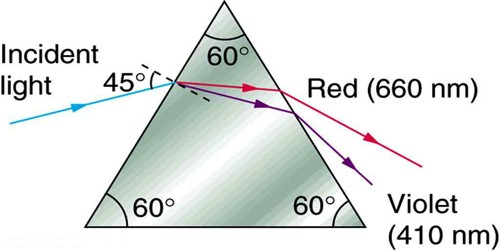Physics

# Magnitude of DispersionMagnitude of Dispersion

We know when a white ray of light passes through a glass prism the emitted ray is splitted into seven colors due to refraction and these colors bend towards the base of the prism. At one end of these colors there exists red color and on the other end, there is violet color. The differences in the angles of deviation between the terminal red and violet rays give the magnitude of dispersion.Fig: angles of deviation of red and violet rays of light

Suppose, δr and δv are respectively the angles of deviation of red and violet rays of light [Figure].

So, dispersion, θ = δv – δr

But the angle of deviation of the mean ray is taken as the angle of deviation of the fundamental ray. The angle of deviation and the dispersion depend on the material of the prism, angle of incidence and angle of the prism. If the prism is placed in a position of minimum deviation, then the angle of deviation of each ray will be minimum.